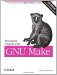# 3.2 Variable Types

There are two types of variables in make : simply expanded variables and recursively expanded variables. A simply expanded variable (or a simple variable) is defined using the := assignment operator:

` MAKE_DEPEND := \$(CC) -M `

It is called "simply expanded" because its righthand side is expanded immediately upon reading the line from the makefile . Any make variable references in the righthand side are expanded and the resulting text saved as the value of the variable. This behavior is identical to most programming and scripting languages. For instance, the normal expansion of this variable would yield:

` gcc -M `

However, if CC above had not yet been set, then the value of the above assignment would be:

` <space>-M `

\$(CC) is expanded to its value (which contains no characters ), and collapses to nothing. It is not an error for a variable to have no definition. In fact, this is extremely useful. Most of the implicit commands include undefined variables that serve as place holders for user customizations. If the user does not customize a variable it collapses to nothing. Now notice the leading space. The righthand side is first parsed by make to yield the string \$(CC) -M . When the variable reference is collapsed to nothing, make does not rescan the value and trim blanks. The blanks are left intact.

The second type of variable is called a recursively expanded variable. A recursively expanded variable (or a recursive variable) is defined using the = assignment operator:

` MAKE_DEPEND = \$(CC) -M `

It is called "recursively expanded" because its righthand side is simply slurped up by make and stored as the value of the variable without evaluating or expanding it in any way. Instead, the expansion is performed when the variable is used . A better term for this variable might be lazily expanded variable, since the evaluation is deferred until it is actually used. One surprising effect of this style of expansion is that assignments can be performed "out of order":

` MAKE_DEPEND = \$(CC) -M ... # Some time later CC = gcc `

Here the value of MAKE_DEPEND within a command script is gcc -M even though CC was undefined when MAKE_DEPEND was assigned.

In fact, recursive variables aren't really just a lazy assignment (at least not a normal lazy assignment). Each time the recursive variable is used, its righthand side is re-evaluated. For variables that are defined in terms of simple constants such as MAKE_DEPEND above, this distinction is pointless since all the variables on the righthand side are also simple constants. But imagine if a variable in the righthand side represented the execution of a program, say date . Each time the recursive variable was expanded the date program would be executed and each variable expansion would have a different value ( assuming they were executed at least one second apart). At times this is very useful. At other times it is very annoying!

#### 3.2.1 Other Types of Assignment

From previous examples we've seen two types of assignment: = for creating recursive variables and := for creating simple variables. There are two other assignment operators provided by make .

The ?= operator is called the conditional variable assignment operator . That's quite a mouth-full so we'll just call it conditional assignment. This operator will perform the requested variable assignment only if the variable does not yet have a value.

` # Put all generated files in the directory \$(PROJECT_DIR)/out. OUTPUT_DIR ?= \$(PROJECT_DIR)/out `

Here we set the output directory variable, OUTPUT_DIR , only if it hasn't been set earlier. This feature interacts nicely with environment variables. We'll discuss this in Section 3.6 later in this chapter.

The other assignment operator, += , is usually referred to as append . As its name suggests, this operator appends text to a variable. This may seem unremarkable, but it is an important feature when recursive variables are used. Specifically , values on the righthand side of the assignment are appended to the variable without changing the original values in the variable . "Big deal, isn't that what append always does?" I hear you say. Yes, but hold on, this is a little tricky.

Appending to a simple variable is pretty obvious. The += operator might be implemented like this:

` simple := \$(simple) new stuff `

Since the value in the simple variable has already undergone expansion, make can expand \$(simple) , append the text, and finish the assignment. But recursive variables pose a problem. An implementation like the following isn't allowed.

` recursive = \$(recursive) new stuff `

This is an error because there's no good way for make to handle it. If make stores the current definition of recursive plus new stuff , make can't expand it again at runtime. Furthermore, attempting to expand a recursive variable containing a reference to itself yields an infinite loop.

` \$  make  makefile:2: *** Recursive variable `recursive' references itself (eventually).  Stop. `

So, += was implemented specifically to allow adding text to a recursive variable and does the Right Thing . This operator is particularly useful for collecting values into a variable incrementally.Managing Projects with GNU Make (Nutshell Handbooks)
ISBN: 0596006101
EAN: 2147483647
Year: 2003
Pages: 131